# What do these word groups have in common

• Slides: 12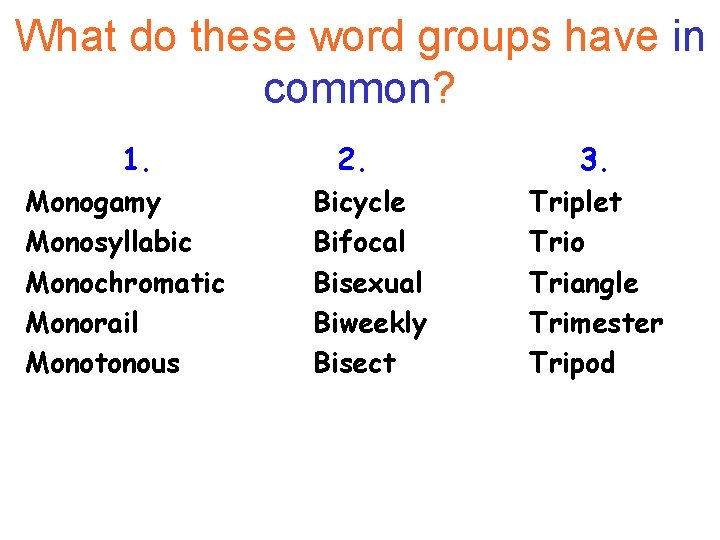What do these word groups have in common? 1. Monogamy Monosyllabic Monochromatic Monorail Monotonous 2. Bicycle Bifocal Bisexual Biweekly Bisect 3. Triplet Trio Triangle Trimester Tripod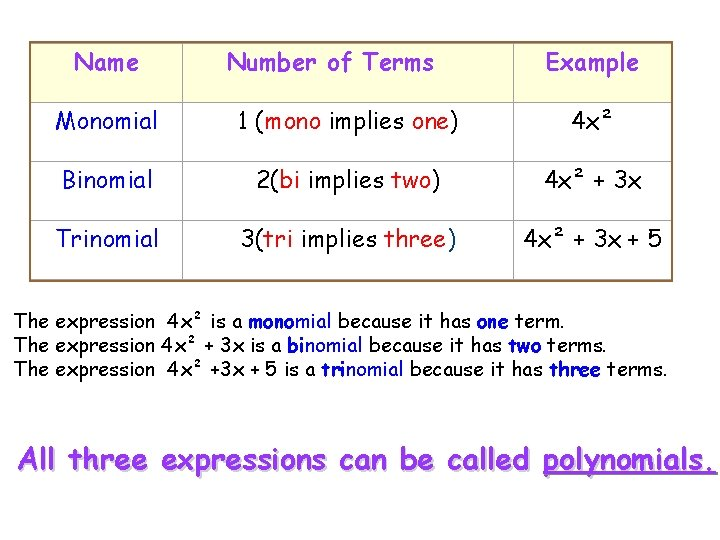Name Number of Terms Example Monomial 1 (mono implies one) 4 x² Binomial 2(bi implies two) 4 x² + 3 x Trinomial 3(tri implies three) 4 x² + 3 x + 5 The expression 4 x² is a monomial because it has one term. The expression 4 x² + 3 x is a binomial because it has two terms. The expression 4 x² +3 x + 5 is a trinomial because it has three terms. All three expressions can be called polynomials.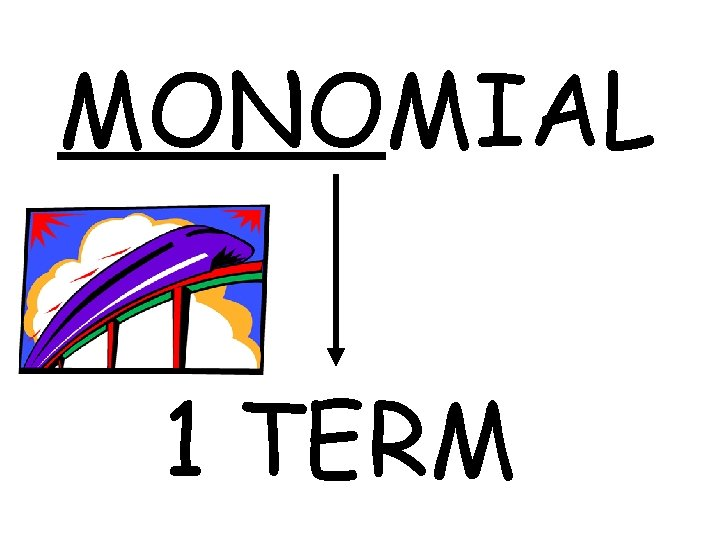MONOMIAL 1 TERM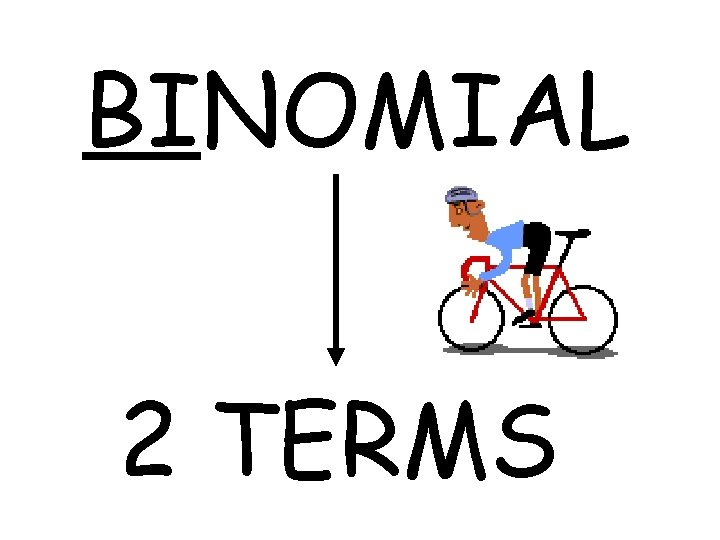BINOMIAL 2 TERMS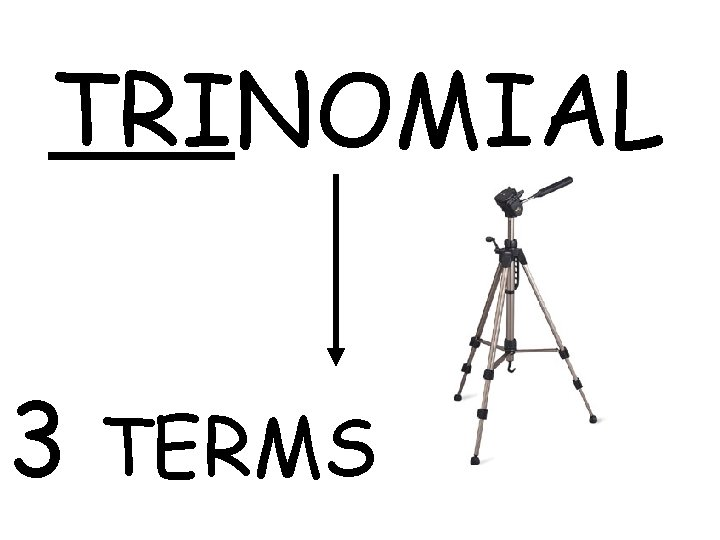TRINOMIAL 3 TERMSAdd: (2 x 2 - 4) + (x 2 + 3 x - 3) MATCH UP LIKE TERMS!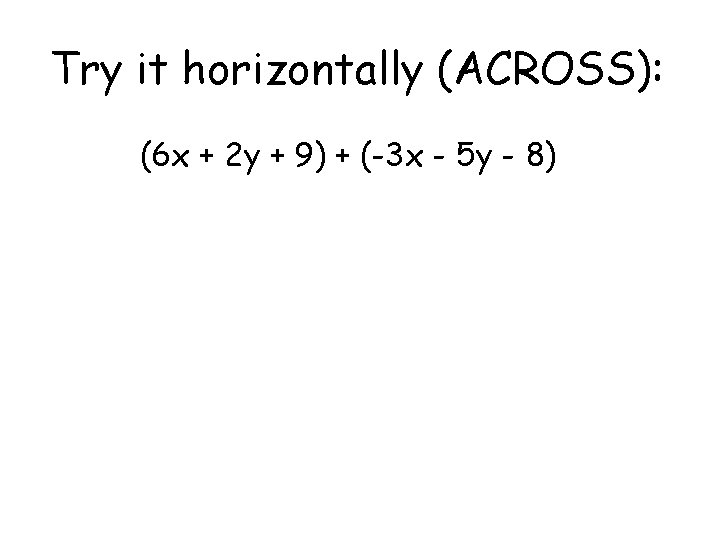Try it horizontally (ACROSS): (6 x + 2 y + 9) + (-3 x - 5 y - 8)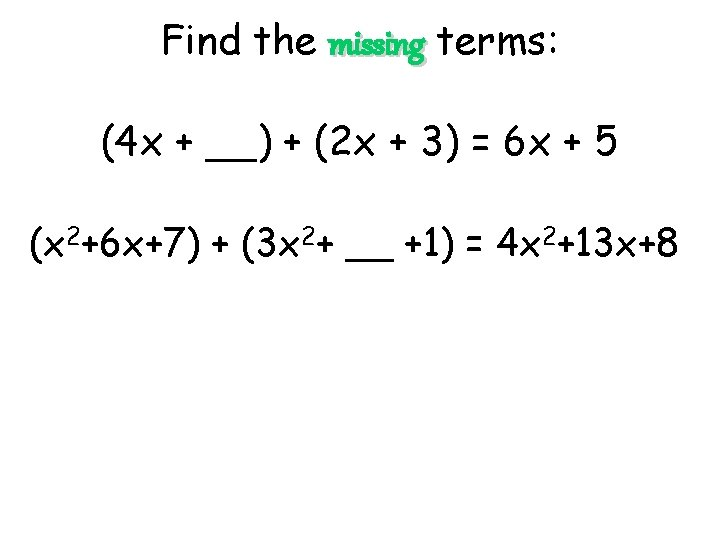Find the missing terms: (4 x + __) + (2 x + 3) = 6 x + 5 (x 2+6 x+7) + (3 x 2+ __ +1) = 4 x 2+13 x+8Simplify: (2 x 2 - 4) - (x 2 + 3 x - 3) Tip: Remember to distribute the NEGATIVE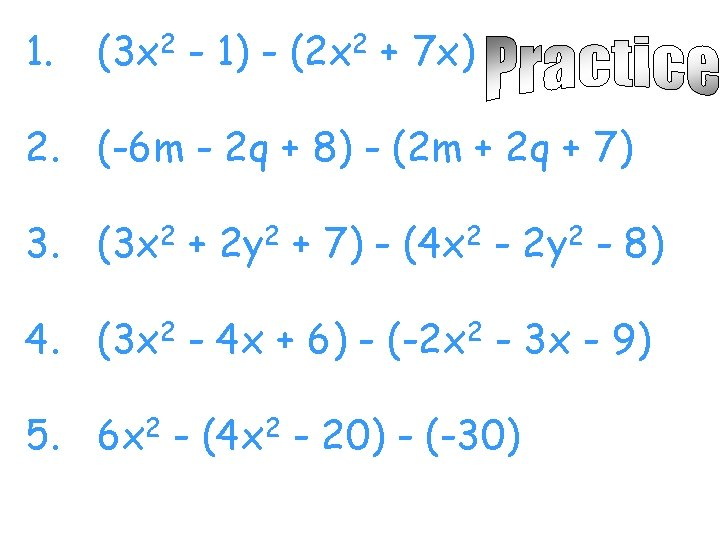1. (3 x 2 - 1) - (2 x 2 + 7 x) 2. (-6 m - 2 q + 8) - (2 m + 2 q + 7) 3. (3 x 2 + 2 y 2 + 7) - (4 x 2 - 2 y 2 - 8) 4. (3 x 2 - 4 x + 6) - (-2 x 2 - 3 x - 9) 5. 6 x 2 - (4 x 2 - 20) - (-30)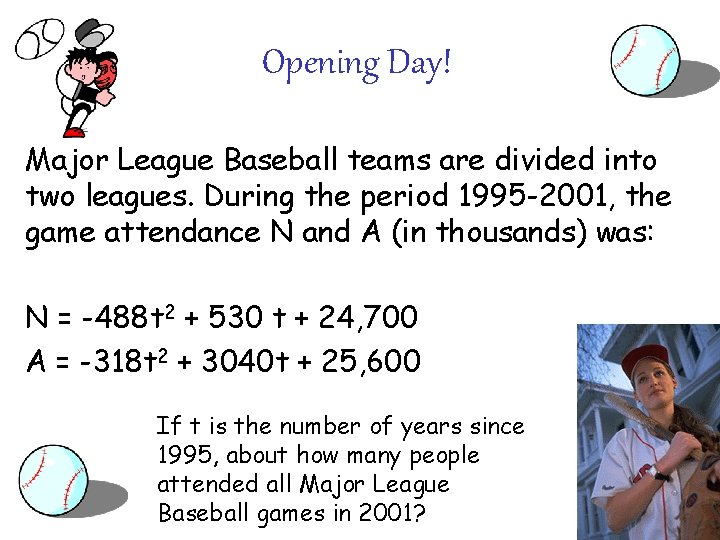Opening Day! Major League Baseball teams are divided into two leagues. During the period 1995 -2001, the game attendance N and A (in thousands) was: N = -488 t 2 + 530 t + 24, 700 A = -318 t 2 + 3040 t + 25, 600 If t is the number of years since 1995, about how many people attended all Major League Baseball games in 2001?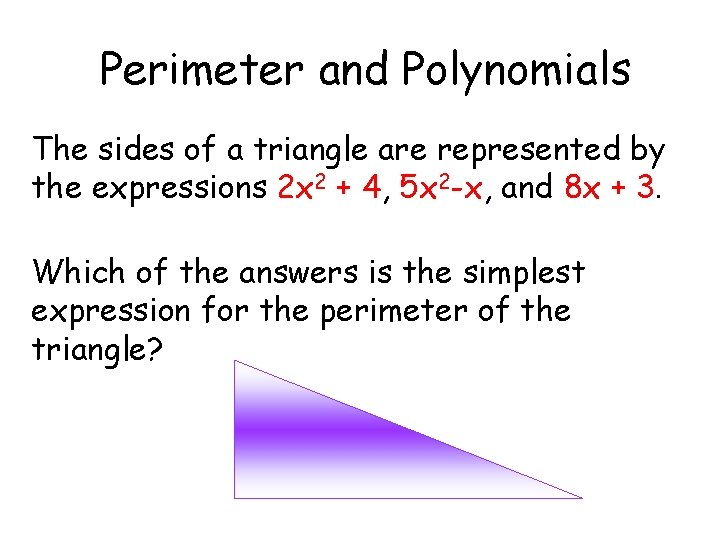Perimeter and Polynomials The sides of a triangle are represented by the expressions 2 x 2 + 4, 5 x 2 -x, and 8 x + 3. Which of the answers is the simplest expression for the perimeter of the triangle?